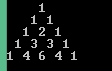## Saturday, 17 January 2015

### C Program to print Pascals Traingle

This is a C Program which prints pascals triangle based on the input given.C Program to print Pascals Triangle

PROGRAM :
```#include <stdio.h>
#include <stdlib.h>

int main()
{
int i,j,x,n,s;
printf("Enter number of lines[height of triangle] : \t");
scanf("%d",&n);
for(i=0; i<=n; i++)
{
x=1;
for(s=1; s<=n-i; s++)
printf(" ");
for(j=1; j<=i+1; j++)
{
printf("%d ",x);
x=x*(i-j+1)/j;
}
printf("\n");
}
return 0;
}```

OUTPUT :C Program to print Pascals Triangle